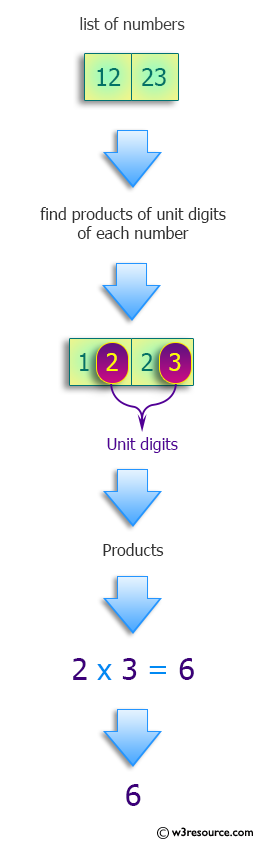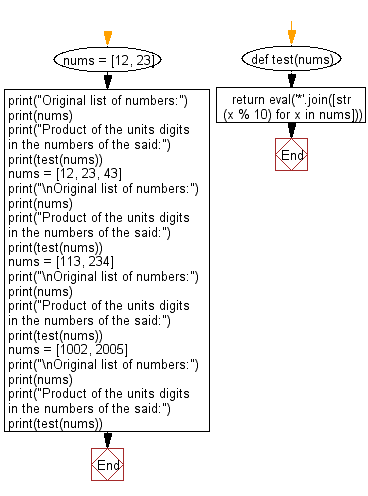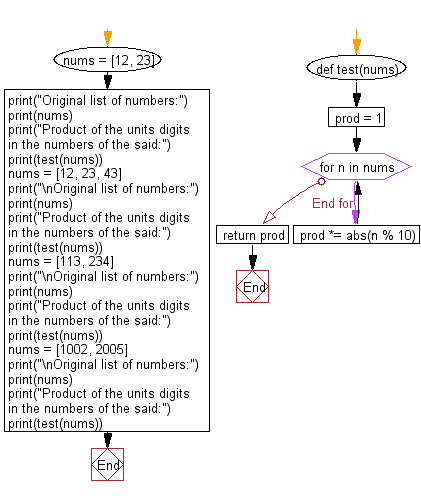﻿ Python: Find the product of the units digits in the numbers - w3resource# Python: Find the product of the units digits in the numbers

## Python Programming Puzzles: Exercise-53 with Solution

Write a Python program to find the product of the units digits in the numbers of a given list.

```Input:
[12, 23]
Output:
6

Input:
[12, 23, 43]
Output:
18

Input:
[113, 234]
Output:
12

Input:
[1002, 2005]
Output:
10
```

Pictorial Presentation:Sample Solution-1:

Python Code:

``````#License: https://bit.ly/3oLErEI

def test(nums):
return eval('*'.join([str(x % 10) for x in nums]))

nums = [12, 23]
print("Original list of numbers:")
print(nums)
print("Product of the units digits in the numbers of the said:")
print(test(nums))
nums = [12, 23, 43]
print("\nOriginal list of numbers:")
print(nums)
print("Product of the units digits in the numbers of the said:")
print(test(nums))
nums = [113, 234]
print("\nOriginal list of numbers:")
print(nums)
print("Product of the units digits in the numbers of the said:")
print(test(nums))
nums = [1002, 2005]
print("\nOriginal list of numbers:")
print(nums)
print("Product of the units digits in the numbers of the said:")
print(test(nums))
``````

Sample Output:

```Original list of numbers:
[12, 23]
Product of the units digits in the numbers of the said:
6

Original list of numbers:
[12, 23, 43]
Product of the units digits in the numbers of the said:
18

Original list of numbers:
[113, 234]
Product of the units digits in the numbers of the said:
12

Original list of numbers:
[1002, 2005]
Product of the units digits in the numbers of the said:
10
```

Flowchart:## Visualize Python code execution:

The following tool visualize what the computer is doing step-by-step as it executes the said program:

Sample Solution-2:

Python Code:

``````#License: https://bit.ly/3oLErEI

def test(nums):
prod = 1
for n in nums:
prod *= abs(n % 10)
return prod

nums = [12, 23]
print("Original list of numbers:")
print(nums)
print("Product of the units digits in the numbers of the said:")
print(test(nums))
nums = [12, 23, 43]
print("\nOriginal list of numbers:")
print(nums)
print("Product of the units digits in the numbers of the said:")
print(test(nums))
nums = [113, 234]
print("\nOriginal list of numbers:")
print(nums)
print("Product of the units digits in the numbers of the said:")
print(test(nums))
nums = [1002, 2005]
print("\nOriginal list of numbers:")
print(nums)
print("Product of the units digits in the numbers of the said:")
print(test(nums))
``````

Sample Output:

```Original list of numbers:
[12, 23]
Product of the units digits in the numbers of the said:
6

Original list of numbers:
[12, 23, 43]
Product of the units digits in the numbers of the said:
18

Original list of numbers:
[113, 234]
Product of the units digits in the numbers of the said:
12

Original list of numbers:
[1002, 2005]
Product of the units digits in the numbers of the said:
10
```

Flowchart:## Visualize Python code execution:

The following tool visualize what the computer is doing step-by-step as it executes the said program:

Python Code Editor :

Have another way to solve this solution? Contribute your code (and comments) through Disqus.

What is the difficulty level of this exercise?

Test your Programming skills with w3resource's quiz.

﻿

## Python: Tips of the Day

Clamps num within the inclusive range specified by the boundary values x and y:

Example:

```def tips_clamp_num(num,x,y):
return max(min(num, max(x, y)), min(x, y))
print(tips_clamp_num(2, 4, 6))
print(tips_clamp_num(1, -1, -6))
```

Output:

```4
-1
```